Courses

# Engineering Mathematics - MCQ Test 1

## 15 Questions MCQ Test Mock Tests Electronics & Communication Engineering GATE 2020 | Engineering Mathematics - MCQ Test 1

Description
This mock test of Engineering Mathematics - MCQ Test 1 for Electronics and Communication Engineering (ECE) helps you for every Electronics and Communication Engineering (ECE) entrance exam. This contains 15 Multiple Choice Questions for Electronics and Communication Engineering (ECE) Engineering Mathematics - MCQ Test 1 (mcq) to study with solutions a complete question bank. The solved questions answers in this Engineering Mathematics - MCQ Test 1 quiz give you a good mix of easy questions and tough questions. Electronics and Communication Engineering (ECE) students definitely take this Engineering Mathematics - MCQ Test 1 exercise for a better result in the exam. You can find other Engineering Mathematics - MCQ Test 1 extra questions, long questions & short questions for Electronics and Communication Engineering (ECE) on EduRev as well by searching above.
QUESTION: 1

Solution:
QUESTION: 2

Solution:
QUESTION: 3

### The order of differential equation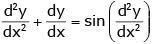Solution:
QUESTION: 4

The order of differential equation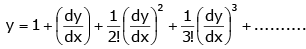Solution:
QUESTION: 5

Find the rank of matrixSolution:
QUESTION: 6

The rank of Diag matrix  [− 1 0 1 0 0 4] is ________

Solution:
QUESTION: 7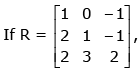the third row of R-1 is

Solution:
QUESTION: 8

For what value of k will the matrix given below become singular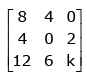is ___________

Solution:

Explanation:-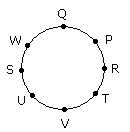QUESTION: 9

The Eigen values of the matrix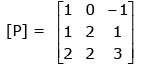Solution:
QUESTION: 10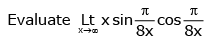Solution:
QUESTION: 11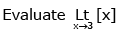Solution:
QUESTION: 12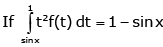then find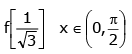Solution:
QUESTION: 13

Given system of linear equations

3x + 2y + z = 0, x + 4y + z = 0, 2x + y + 4z = 0, has

Solution:
QUESTION: 14

Suppose that the probability of event A is 0.2 and the probability of event B is 0.4. Also, suppose that the two events are independent. Then P(A|B) is:

Solution:
QUESTION: 15

The Newton-Raphson method formula for finding the square root of a real number R from the equation x2 − R = 0 is

Solution: